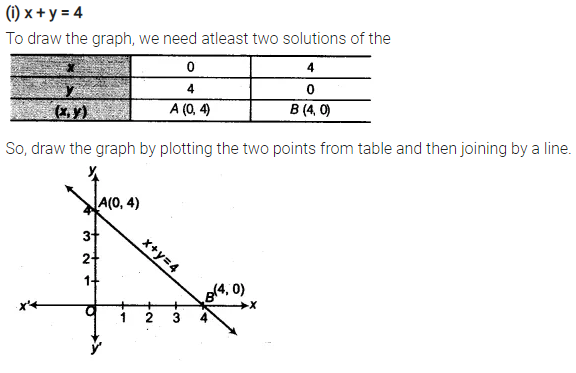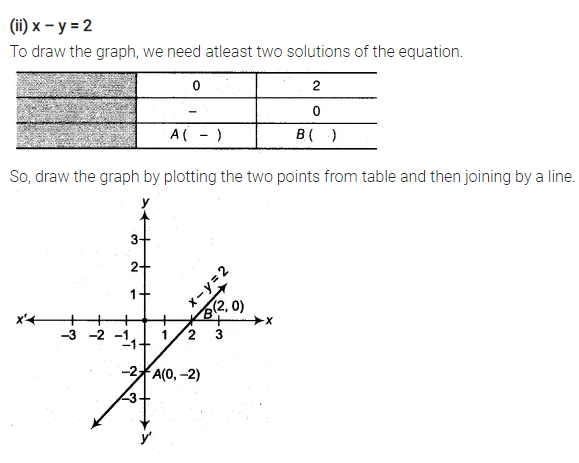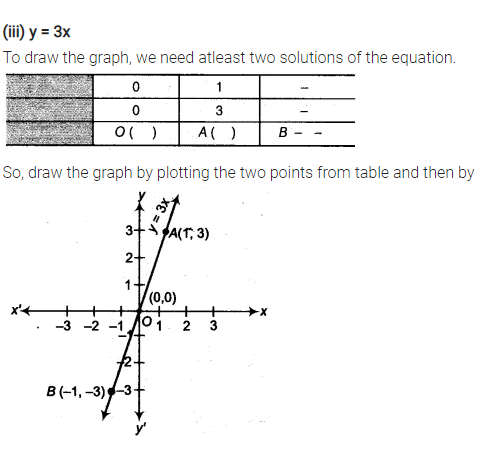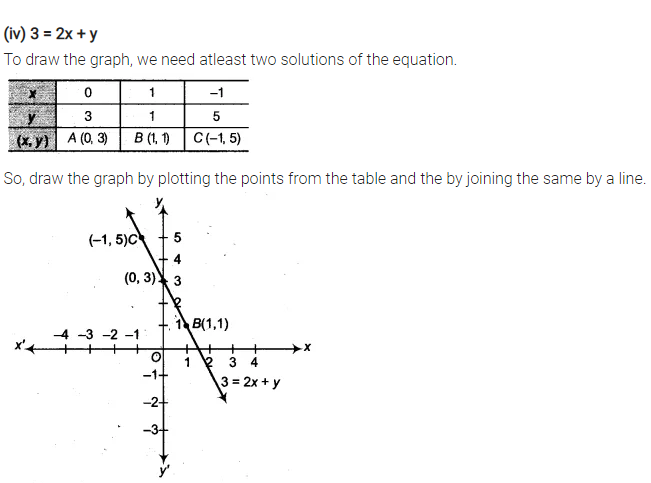# Draw the graph of each of the following linear equations in two variables

Draw the graph of each of the following linear equations in two variables
(i) x + y = 4
(ii) x – y = 2
(iii) y = 3x
(iv) 3 = 2x + y